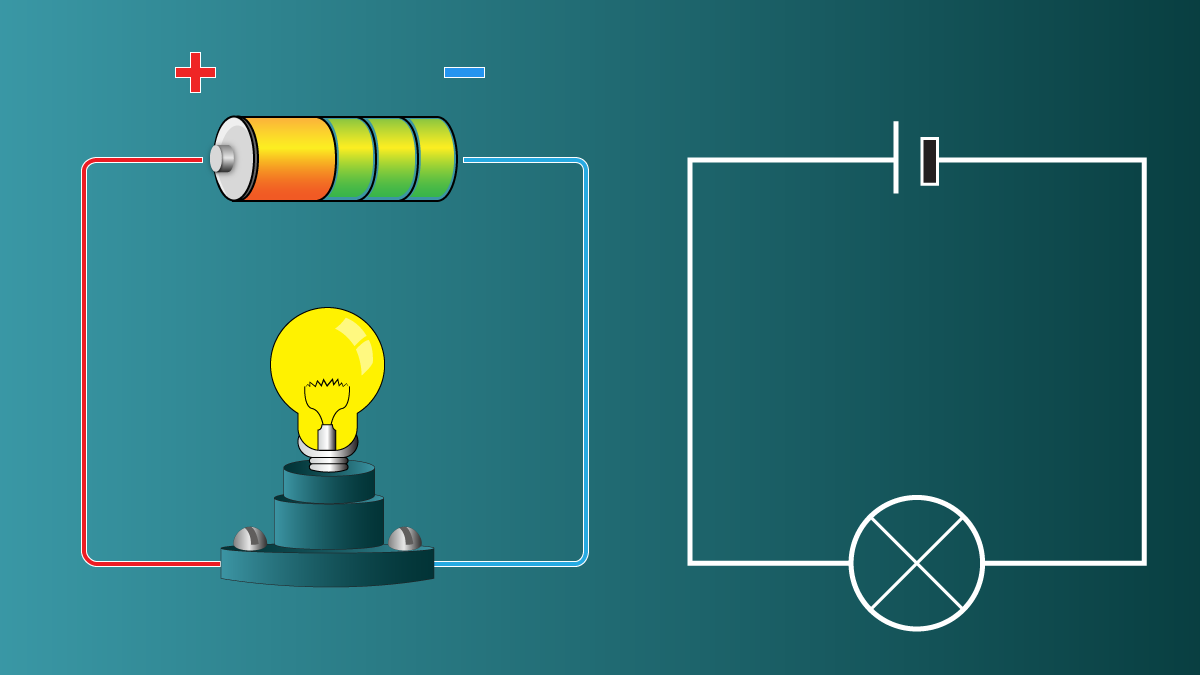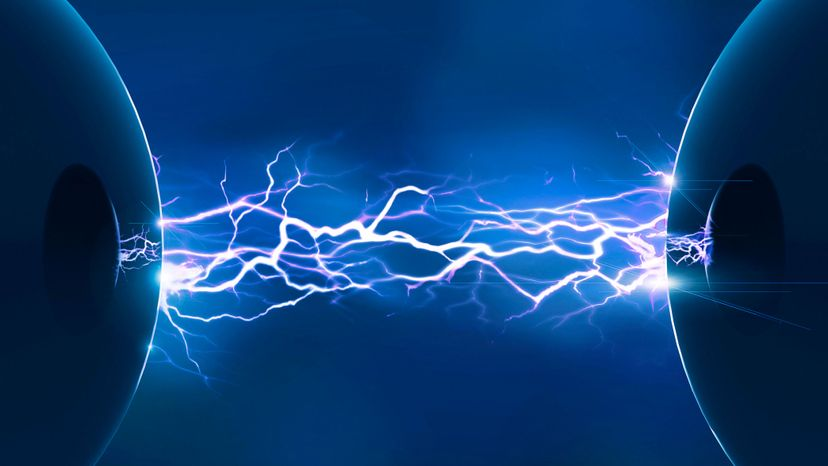Free shipping within Continental USA on orders over \$100. 10% off first purchase for joining our news letter. 5x in loyalty points on product price and free gift if purchase is over \$500.

# Amps, Volts, Watts what do these mean in relation to power generation?Calculating the power of electricity involves considering several key factors that determine the amount of electrical energy consumed or produced in an electrical circuit. The power of electricity is typically measured in watts (W) or kilowatts (kW), and it represents the rate at which electrical energy is used or generated. The main factors that go into calculating the power of electricity are:1. Voltage (V): Voltage, also known as electric potential difference, is the force that drives electric charges through a circuit. It is measured in volts (V). The power in an electrical circuit is directly proportional to the voltage. Higher voltage levels result in higher power consumption or production.
2. Current (I): Current refers to the flow of electric charges through a circuit and is measured in amperes (A). The power in an electrical circuit is directly proportional to the current. Higher current levels indicate higher power consumption or production.
3. Power Factor (PF): Power factor is a dimensionless number that represents the efficiency of an electrical system in converting electrical power into useful work. It is the ratio of real power (measured in watts) to apparent power (measured in volt-amperes). A power factor of 1 (or unity) indicates perfect efficiency, while a power factor less than 1 indicates some inefficiencies in the system due to reactive power consumption.The formula for calculating the power in an electrical circuit is:

Power (P) = Voltage (V) × Current (I) × Power Factor (PF)

In direct current (DC) circuits, the power factor is typically equal to 1, as there is no reactive power involved. Therefore, the power calculation simplifies to:

Power (P) = Voltage (V) × Current (I)

In alternating current (AC) circuits, the power factor becomes a crucial factor to consider, especially in large-scale electrical systems where reactive power (due to inductive or capacitive loads) can significantly affect the overall power consumption and efficiency.

1. Resistance (R): In some cases, particularly in DC circuits or resistive AC loads, electrical power can also be calculated using Ohm's law. Ohm's law states that the current (I) flowing through a resistor (R) when a voltage (V) is applied across it is given by:

I = V / R

By substituting this value of current (I) into the power formula, we get:

Power (P) = V^2 / R

where V is the voltage and R is the resistance.

1. Impedance (Z): In AC circuits or circuits with reactive elements (e.g., inductors and capacitors), impedance (Z) is used instead of resistance. Impedance is the total opposition offered to the flow of alternating current and is measured in ohms (Ω). The relationship between voltage (V), current (I), and impedance (Z) in an AC circuit is represented by the equation:

I = V / Z

The power calculation for AC circuits with impedance is similar to the formula for resistance:

Power (P) = V^2 / ZIn summary, the main factors that go into calculating the power of electricity are voltage, current, and power factor. For DC circuits or resistive loads, resistance is also a key factor. In AC circuits with reactive elements, impedance plays a crucial role in determining power consumption or production. Understanding these factors is essential for analyzing electrical systems, designing efficient electrical networks, and managing power consumption effectively.